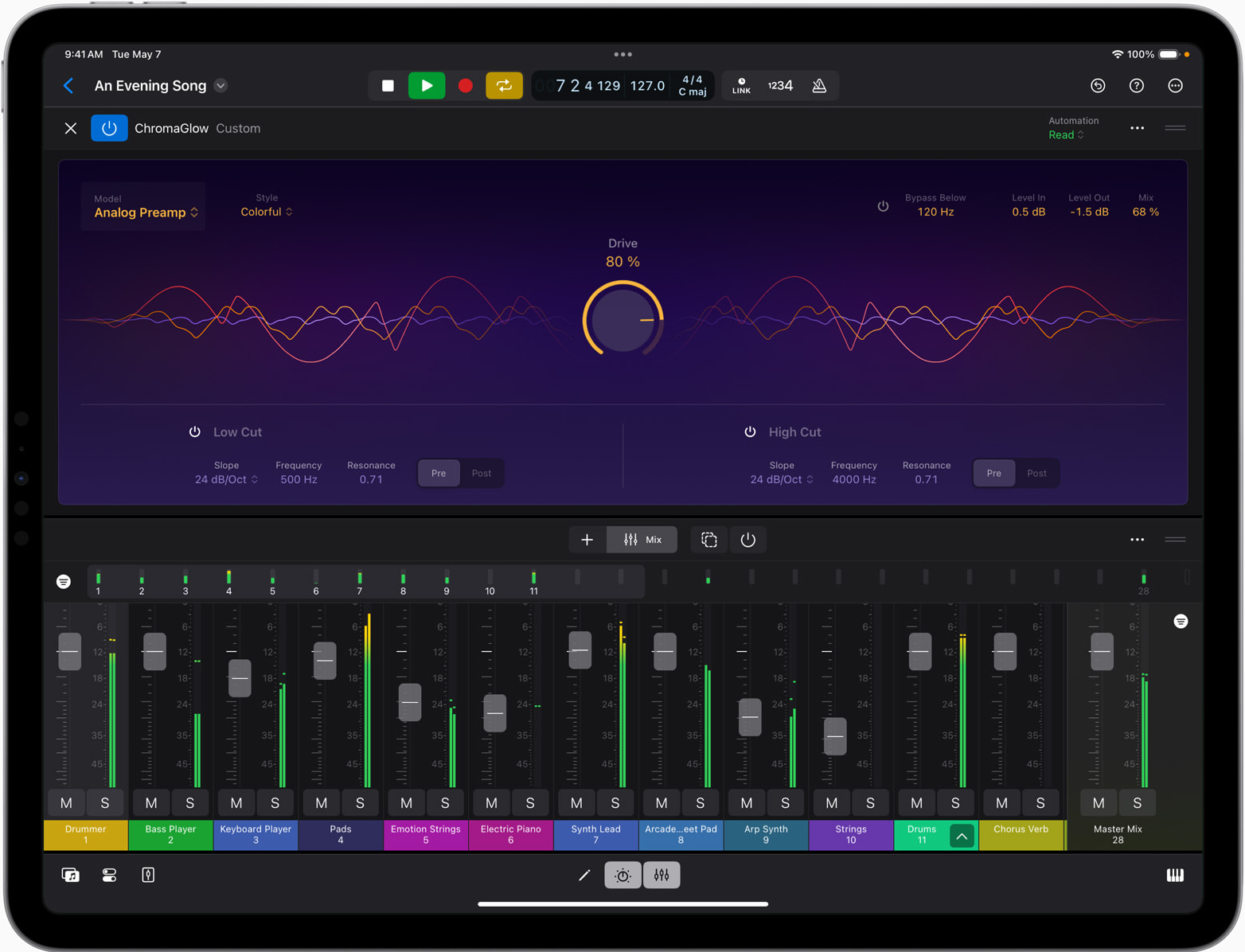# Accelerate

The Accelerate framework provides high-performance, energy-efficient computation on the CPU by leveraging its vector-processing capability. Accelerate performs optimized large-scale mathematical computations and image calculations so you can write apps that leverage machine learning, data compression, signal processing, and more.### Machine Learning

The Accelerate framework’s BNNS library is a collection of functions that you use to construct neural networks for training and inference. The library provides routines optimized for high performance and low-energy consumption across all CPUs that the macOS, iOS, tvOS, and watchOS platforms support. BNNS includes a rich set of layer types, loss functions, activation functions, and supporting subroutines for machine learning.

### Image Processing

vImage is a high-performance, image-processing framework. It includes functions for image manipulation—convolutions, geometric transformations, histogram operations, morphological transformations, and alpha compositing—as well as utility functions for format conversions and other operations.

vImage optimizes image processing by using the CPU’s vector processor. If a vector processor is not available, vImage uses the next best available option. This framework allows you to reap the benefits of vector processors without having to write vectorized code.

### Digital Signal Processing

The vDSP framework contains a collection of highly optimized functions for digital signal processing and general purpose arithmetic on large arrays. Examples of digital signal-processing functions are Fourier transform and biquadratic filtering operations. Arithmetic functions include multiply-add and reduction functions, such as sum, mean, and maximum.

### Vector and Matrix Computation

With vForce, you can perform arithmetic and transcendental functions on vectors. Because they are vectorized functions, vForce operations are significantly faster and more energy-efficient than performing the same operations in loops over the same vectors.

The simd library provides types and functions for small-vector and small-matrix computations. The types include integer and floating-point vectors and matrices. The functions provide basic arithmetic operations, element-wise mathematical operations, and geometric and linear algebra operations.

simd supports vectors that contain up to 16 elements (for single-precision values) or 8 elements (for double-precision values), and matrices up to 4 x 4 elements in size.

### Linear Algebra

The Accelerate framework provides BLAS and LAPACK libraries for performing linear algebra on dense vectors and matrices. Accelerate's BLAS and LAPACK implementations abstract the processing capability of the CPU so code written for them will execute the appropriate instructions for the processor available at runtime. This means that both BLAS and LAPACK are optimized for high performance and low-energy consumption.

BLAS contains the linear algebra primitives, including vector-vector, matrix-vector, and matrix-matrix operations. LAPACK includes support for eigenvalue and singular-value problems, matrix factorization, as well as solving systems of linear equations and linear least squares.

### Lossless Compression

AppleArchive provides fast compression that includes file attributes, such as, ownership, permissions, flags, times, extended attributes, and error correction. AppleArchive offers these features:

• Multithreaded processing that uses all cores, is energy efficient, and yields faster results
• An ability to transport files and their attributes and use Apple File System (APFS) features when they’re available, for example, filesystem compression, full clones, and sparse files
• Flexible encoding formats, so you can use archives, for example, for error correction, digests, manifests, and external data storage
• API support for in-memory archive processing, streaming access, random access, and back-to-back archive and extraction

### Spatial

Spatial is a lightweight 3D mathematical library that provides a simple API for working with 3D primitives. It includes 3D point, size, and rectangle primitives; and affine and projective transforms. Much of its functionality is similar to the 2D geometry support in Core Graphics, but in three dimensions. Because Spatial is build on simd, it offers high performance 3D operations.

### Sparse Solvers

Using the Sparse Solvers library in the Accelerate framework, you can perform linear algebra on systems of equations where the coefficient matrix is sparse, that is, most of the entries in the matrix are zero.

Many problems in science and technology require the solution of large systems of simultaneous equations. When these equations are linear, they normally appear as the matrix equation Ax = b (and even when the equations are nonlinear, solving the problem is often a sequence of linear approximations).